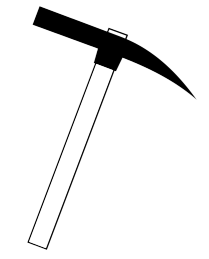## 7. A Sum of Numbers

Reminder: a natural number is a positive whole number.

The squares of natural numbers can be calculated in one quite a peculiar manner. It can be demonstrated that each square of a natural number n is equal to the sum of the first n odd numbers. For example:

```1×1 =  1 = 1
2×2 =  4 = 1+3
3×3 =  9 = 1+3+5
4×4 = 16 = 1+3+5+7
```

That this property of squares is true can be shown by a geometric demonstration:This square represents the number 16, as it is composed of 16 parts. It is a square of a number 4. The number of parts in each shade of grey equals one of the odd numbers 1, 3, 5, 7.

Let us now write a program that writes out the first 10 squares by using this method. If we were to take a moment and think about the program we are about to write, it would become apparent that the program has to calculate consecutive odd numbers. We already know how to add them up.

```// A program that calculates and prints out the first ten squares.

#include <iostream>
using namespace std;

int main()
{
double sum = 0;
double oddNumber = 1;        // First odd number is 1
for(int i=1; i<=10; i++)
{
sum += oddNumber;
cout << sum << endl;
oddNumber += 2;          // Calculate the next odd number
}
}
```

There is something obviously new in the text of this program, namely, in the lines containing the symbols '`//`'. This is the means of denoting comments in the C++ language. A comment is a part of the code that will be ignored during translation by a compiler. A comment's sole purpose is to convey additional information to the reader of the program.

The symbols '`//`' begin a comment that spans until the end of the line. The entire text on the right-hand side of symbols '`//`' will be considered as a comment by a compiler.

You surely remember what the `+=` operator does, right?Analyze this program by yourself to figure out how it works. Use a debugger! Also use a debugger to execute this program step by step at least once.

Let us write this program in another manner:

```/* A second program that calculates and prints out the first ten squares.
It calculates odd numbers by using a formula .
*/

#include <iostream>
using namespace std;

int main()
{
double sum = 0;
double oddNumber;
for(int i=1; i<=10; i++)
{
/* The next statement calculates the i-th odd number by a formula */
oddNumber = i*2 - 1;
sum += oddNumber;
cout << sum << endl;
}
}
```

There is another type of a comment at the beginning of this program. Anything enclosed between symbols '`/*`' and '`*/`' is a comment in the C and C++ languages. This kind of a comment can span over multiple lines.

Execute this program by a computer. Then do it step by step with a debugger.

Of course, the simplest way to calculate a square would be by multiplying a number by itself, but the two programs given demonstrate how the squares can also be calculated by adding up odd numbers.